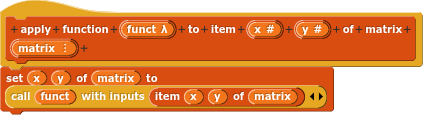# Matrixes Extension (V2)

Matrices Extension, By: jacobmorrison

Blocks:

Matrix _ _ (creates a matrix),

Set _ _ of _ to _ (set (x,y) of the matrix to given value),

item _ _ of _ (get an item of matrix at (x,y)),

append front _ to _ at _ _ (append value in front of (x, y) in matrix),

append back _ to _ at _ _ (append value behind (x,y) in matrix),

for each item in matrix _: (for item in matrix),

remove _ _ of _ (remove item at (x,y) of matrix),

rows of matrix _, (get rows of matrix)

columns of matrix _, (get columns of matrix)

append row to _, (add a row to a matrix)

append column to _, (add a column to a matrix)

remove row of _, (remove a row of a matrix)

remove column of _ (remove a column of a matrix)

point _ _ exists in matrix _?, (return if point (x, y) exists in matrix)

apply function _ to item _ _ in matrix _ (calls function on item (x, y) in matrix, passing item (x, y) of matrix as a parameter)

fill from _ _ to _ _ in matrix _ with _ (fills from x1, y1 to x2, y2 in matrix with item)

Hi, welcome to the forum!

Matrix support is a good idea, but you should check out the matrix support that's already in Snap!. For example, your ROWS OF MATRIX could just use the primitive LENGTH OF instead of looping through all the items. (And COLUMNS OF is just LENGTH OF (ITEM 1 OF).)

I was confused by your APPEND blocks because I expected them to add new items rather than extending the text in an item. That seems like an extremely special-purpose operation. It'd be better, I think, if you generalized that to

Also, when people want to remove a row or column of a matrix, it isn't always the last one. Think about computing the determinant of a matrix as an example.

Thanks! I am very new to Snap! and coding in general, so I don't really know how to do this as efficiently as possible. However, I will update this to accommodate the recommendations you gave. Thanks!

Matrix multiplication(?)

I've done a few blocks like this (but not as comprehensively as you've done) and then I forget which project they are in so it'll be nice to be able to just do a search on here and find a libraryI'd like to ask the more advanced Snappers to not jump in and say - use this script insteadBut just give hints like Brian has, so that the OP can learn and grow with Snap!My first hint would be to see if you can use the reshape reporter to simplify and speed up the matrix reporter

I refrained myself from doing that when I first saw this topic.

1. What do you mean by Snap!'s built-in matrix support? I know that If you put a list in a list, it will show up as a matrix, this is how I made the matrix block.

2. With the apply function block, should the function take the item at (x, y) of (matrix) as an argument?

Thanks again for the help!

Most scalar functions, e.g., arithmetic functions that ordinarily take individual numbers as inputs, can be applied to vectors and matrices, in which case they apply themselves termwise to the underlying numbers:

Mixing a scalar and an array input combines the scalar with each item of the array:

Similarly, you can combine a vector with a matrix if the length of the vector equals the length of a row of the matrix.

Notice that we always do termwise matching, which is right for adding vectors or adding matrices, and is right for multiplying a vector by a scalar. But the termwise product of two vectors is neither their dot product (which is the sum of all the items in the termwise product) nor their cross product (which is a vector perpendicular to the two input vectors in 3-space). And the termwise product of two matrices is nothing like their mathematical product.

If you load the APL library, then you get a very extensive set of mathematically correct operations.

And yes, APPLY FUNCTION is meant as a generalization of your JOIN block. Note that the features described above eliminate much of the need for APPLY FUNCTION because most scalar functions automatically extend themselves to vectors and matrices (and higher-dimensional arrays, too). But occasionally you'll need it for non-primitive functions that don't automatically extend in that way, usually because they include an IF/ELSE operation.

Just so that I am understanding this correctly, is this how the function should work?Yup!!

Sorry, but most matrix operations will take a while because I don't really know about them. However, addition and subtraction should work with the built-in (n1)+(n2) and (n1)-(n2) reporters.

im scrolling through on my phone rn so im gonna wait till i get home to check out your project but just want to say, not sure if you've had a good chance to experiment with the map, keep, and combine blocks yet, but they are very versatile and extendable for these kinds of things, i think youd do well tinkering around with those concepts might sparks some new ideas and more optimized ways of thinking.

Thanks! As I said earlier, I am fairly new to Snap! and coding in general, so I had no idea those even existed. I will check them out though. Thanks!

That's Cool!
(and I said that because it really is cool)
(my username has nothing to do with this message)

I really like owls, somebody should make an owl game
(my username has nothing to do with this message)